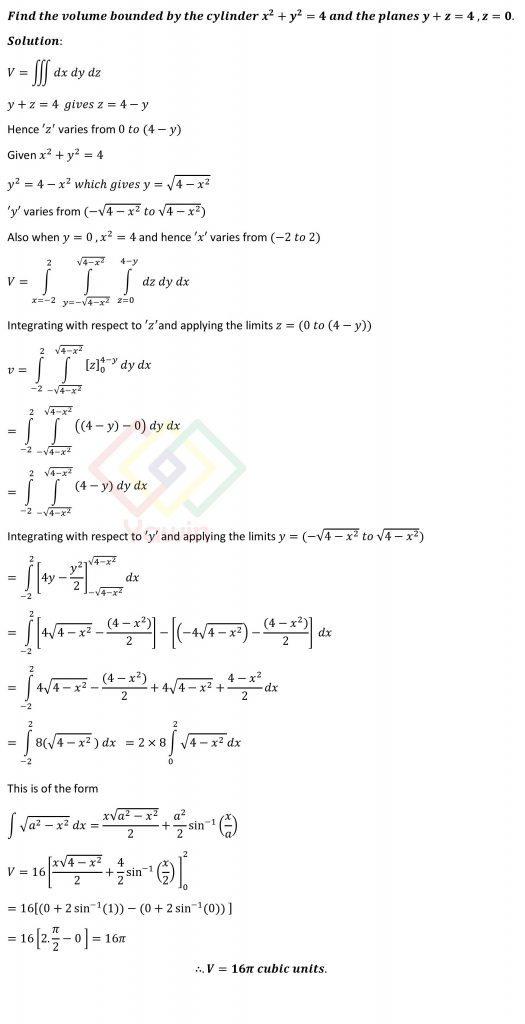Find the volume bounded by the cylinder x^2+y^2=4 and the planes y+z=4, z=0

## Question## Question

Find the volume bounded by the cylinder x^2+y^2=4 and the planes y+z=4, z=0

## Topic

Applications of Double Integrals and Triple Integrals